UPSC  >  NCERT Solutions: Motion & Time

# NCERT Solutions: Motion & Time - Notes | Study Science & Technology for UPSC CSE - UPSC

 1 Crore+ students have signed up on EduRev. Have you?

Question: 1. Classify the following as motion along a straight line, circular or oscillatory motion:

(i) Motion of your hands while running.

(ii) Motion of a horse pulling a cart on a straight road.

(iii) Motion of a child in a merry-go-round.

(iv) Motion of a child on a see-saw.

(v) Motion of the hammer of an electric bell.

(vi) Motion of a train on a straight bridge.

(i) Periodic motion or Oscillatory motion

(ii) Along a straight line (Linear motion)

(iii) Circular motion

(iv) Periodic motion or oscillatory motion

(v) Periodic motion or oscillatory motion

(vi) Linear motion

Question: 2. Which of the following are not correct?

(i) The basic unit of time is second.

(ii) Every object moves with a constant speed.

(iii) Distances between two cities are measured in kilometres.

(iv) The time period of a given pendulum is not constant.

(v) The speed of a train is expressed in m/h.

Question: 3. A simple pendulum takes 32 s to complete 20 oscillations. What is the time period of the pendulum?

Given, Number of oscillation = 20

Time taken = 32 second

We know that,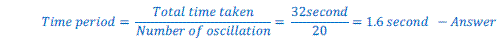Question: 4. The distance between two stations is 240 km. A train takes 4 hours to cover this distance. Calculate the speed of the train.

Answer: Given, distance = 240 km

Time taken = 4 hour

We know that,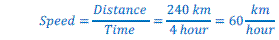Question: 5. The odometer of a car reads 57321.0 km when the clock shows the time 08:30 AM. What is the distance moved by the car, if at 08:50 AM, the odometer reading has changed to 57336.0 km? Calculate the speed of the car in km/min during this time. Express the speed in km/h also.

Given,

Initial reading of odometer = 57321.0 km

Final reading of odometer = 57336.0 km

Initial time = 08:30 AM

Final time = 08:50 AM

Thus,

Distance covered = Final reading of odometer – Initial reading of odometer

= 57336.0 km – 57321.0 km = 15 km

Total time taken = Final time – Initial time = 08:50 AM – 08:30 AM = 20 minute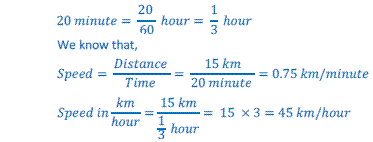So, speed = 0.75 km/minute or 45km/hour

Question: 6. Salma takes 15 minutes from her house to reach her school on a bicycle. If the bicycle has a speed of 2 m/s, calculate the distance between her house and the school.

Given :
Speed of the bicycle= 2 m/s
Time taken by Salma to reach school from her house = 15 minutes = 15 × 60 s = 900 s
We know that speed = distance / time
Hence Distance = Speed × time
= 2 m/s × 900 sec
= 1800 m
=1.8 km
Distance between Salma's house and school =1.8 km

Question: 7. Show the shape of the distance-time graph for the motion in the following cases:

(i) A car moving with a constant speed.

(ii) A car parked on a side road.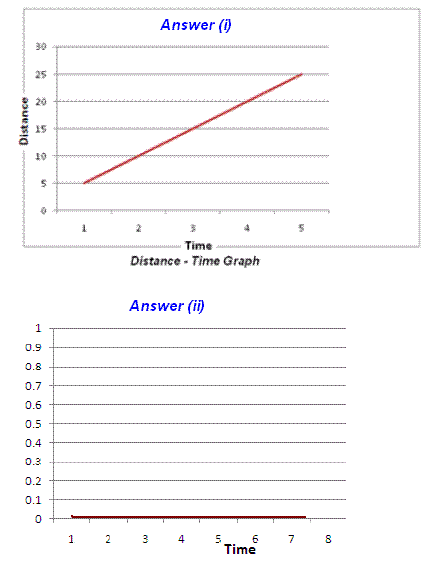Question: 8. Which of the following relations is correct?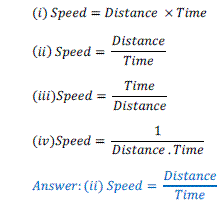Question: 9. The basic unit of speed is:

(i) km/min

(ii) m/min

(iii) km/h

(iv) m/s

Question: 10. A car moves with a speed of 40 km/h for 15 minutes and then with a speed of 60 km/h for the next 15 minutes. The total distance covered by the car is:

(i) 100 km

(ii) 25 km

(iii) 15 km

(iv) 10 km

Given,

Speed for first 15 minute = 40km/h

Speed for next 15 minute = 60 km/h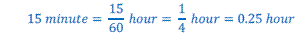We know that,

Distance = Speed * Time

Therefore, Distance covered in first 15 minute i.e. 0.25 hour = 40 km/h * 0.25 hour = 10 km

Distance covered in next 15 minute = 60 km/h * 0.25 hour = 15 km

Thus, total distance covered = 10 km + 15 km = 25 km

Question: 11. Suppose the two photographs, shown in Fig. 13.1 and Fig. 13.2 had been taken at an interval of 10 seconds. If a distance of 100 metres is shown by 1 cm in these photographs, calculate the speed of the blue car.

Figure in question is given from text book.

Do the following steps to calculate the speed:

Measure the distance cover by blue car.

Multiply the distance measured in cm by 100 to get the distance covered by car in meter.

Divide th distance in meter by 10 second (time given in question).

Result will be the speed of car in meter/second.

Suppose the distance of car is 3.5 cm.

Thus, Distance covered by car = 3.5 x 100 = 350 meter.

Time (given in question) = 10 second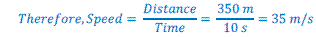Question: 12. Figure given here shows the distance-time graph for the motion of two vehicles A and B. Which one of them is moving faster?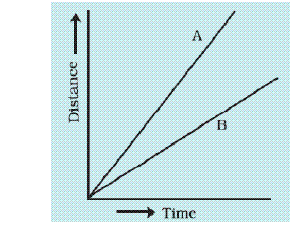Answer: Vehicle A is moving faster which is evident from higher slope for distance time graph of this vehicle; compared to that of the other vehicle.

Question: 13. Which of the following distance-time graphs shows a truck moving with speed which is not constant?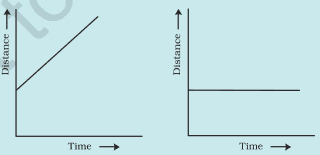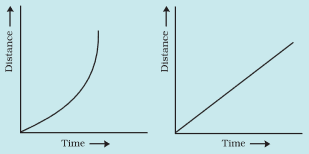Answer: (iii) The slope of the graph in this option is not a straight line and hence it does not show a uniform motion.

The document NCERT Solutions: Motion & Time - Notes | Study Science & Technology for UPSC CSE - UPSC is a part of the UPSC Course Science & Technology for UPSC CSE.
All you need of UPSC at this link: UPSC

## Science & Technology for UPSC CSE

86 videos|352 docs|235 tests

## Science & Technology for UPSC CSE

86 videos|352 docs|235 tests

### How to Prepare for UPSC

Read our guide to prepare for UPSC which is created by Toppers & the best Teachers

Track your progress, build streaks, highlight & save important lessons and more!

,

,

,

,

,

,

,

,

,

,

,

,

,

,

,

,

,

,

,

,

,

;